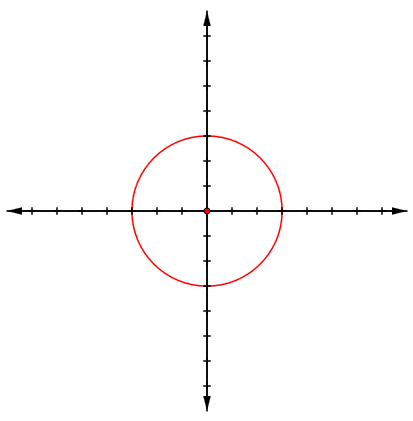### Home > GC > Chapter 12 > Lesson 12.1.1 > Problem12-9

12-9.

On graph paper, graph $x^2 + y^2 = 9$.1. Consider the inequality $x^2 + y^2 \le 9$. Does the point $\left(0,0\right)$ make this inequality true? What is the graph of $x^2 + y^2 \le 9$? Explain.
2. Now consider the inequality $x^2+y^2 > 9$. Does the point $\left(0,0\right)$ make this inequality true? What region is shaded? Describe the graph of this inequality.